Next: A Matching Algorithm Up: Finding Good Matchings Previous: Finding Good Matchings

## Matching Criteria for Keyless Data

Our goal in this section is to augment the trivial label-matching criterion with additional criteria that simultaneously yield matchings that are meaningful in the domains of the data being considered, and that make possible efficient algorithms to compute a best matching.

Our first matching criterion states that nodes that are ``too dissimilar'' may not be matched with each other. For leaf nodes, this condition is stated as follows.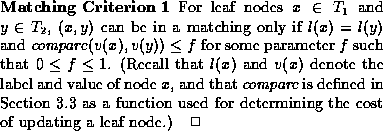We also want to disallow matching internal nodes that do not have much in common. Here a more natural notion than the value (which is often null in the label-value model) is the number of common descendants. Let us say that an internal node x contains a node y if y is a leaf node descendent of x, and let |x| denote the number of leaf nodes x contains. The following constraint allows internal nodes x and y to match only if at least a certain percentage of their leaves match (where t is a parameter):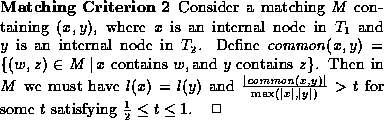Recall from Section 1 that one of the features of our work is that we use domain characteristics to design efficient algorithms. We now introduce these domain characteristics and formalize them by stating two assumptions that they let us make.

The hierarchical keyless data we are comparing has labels, and these labels usually follow a structuring schema, such as the one defined in [ACM95]. Many structuring schemas satisfy an acyclic labels condition, formalized in the following assumption: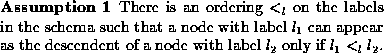In schemas where this condition is not satisfied, we can use domain semantics to merge labels that form a cycle, so that the resulting schema satisfies this condition [CRGMW95].

Our next assumption states (informally) that the compare function is a good discriminator of leaves. It states that given any leaf s in the old document, there is at most one leaf in the new document that is ``close'' to s, and vice versa. For example, consider a world-wide web ``movie database'' source listing movies, actors, directors, etc. A tree representation of this data may contain movie titles as leaves. This assumption says that, when comparing two snapshots of this data, a movie title in one snapshot may ``closely resemble'' at most one movie title in the other.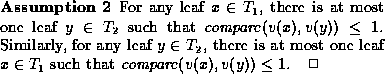This assumption may not hold for some domains. For example, legal documents may have many sentences that are almost identical. The algorithms we describe below are guaranteed to produce an optimal matching when Assumption 2 holds. When Assumption 2 does not hold, our algorithm may generate a suboptimal, but still correct, solution. However, we can often post-process such a suboptimal solution to obtain an optimal solution. We discuss this issue further in Section 6.3.

Matching Criteria 1 and 2 and the assumptions that we have introduced above allow us to simplify the best matching problem as follows. (Recall that a best matching is a matching that can be used to produce an edit script of the lowest cost among all matchings satisfying the Matching Criteria.) We say that a matching is maximal if it is not possible to augment it without violating the Matching Criteria. We can show that our Matching Criteria imply that there is a unique maximal matching. Furthermore, given our assumptions, we can show that this unique maximal matching is also the best matching. These statements are formalized in the following theorem, which is proven in [CRGMW95]: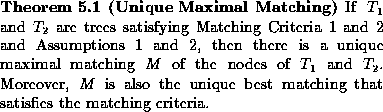Next: A Matching Algorithm Up: Finding Good Matchings Previous: Finding Good Matchings

Sudarshan S. Chawathe
Wed Jun 19 08:22:34 PDT 1996